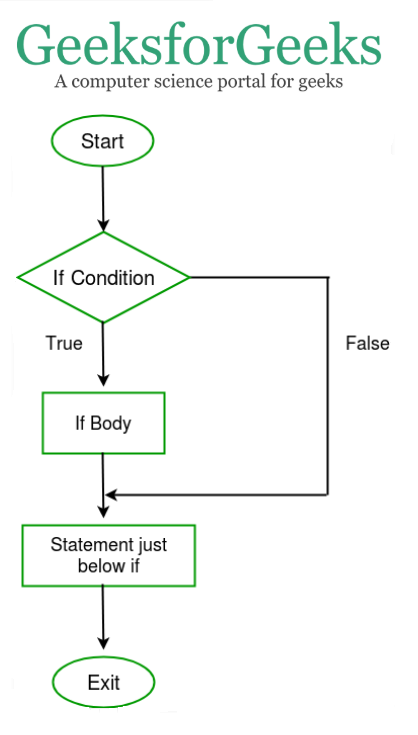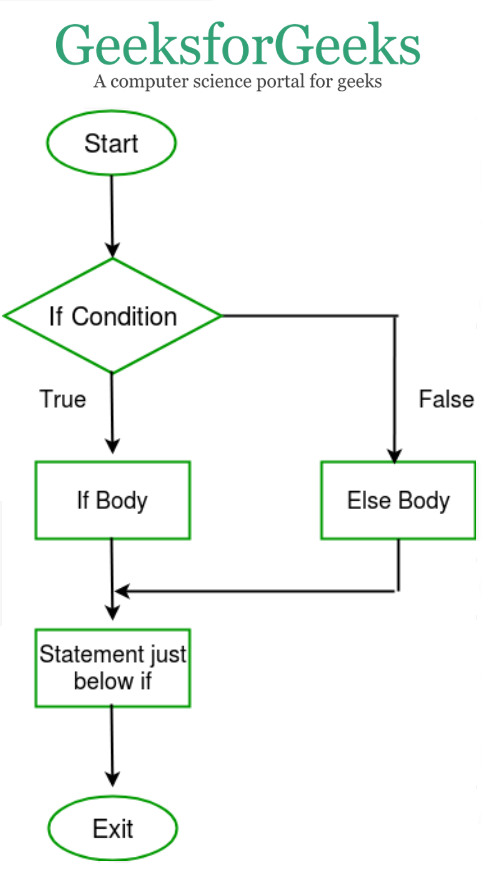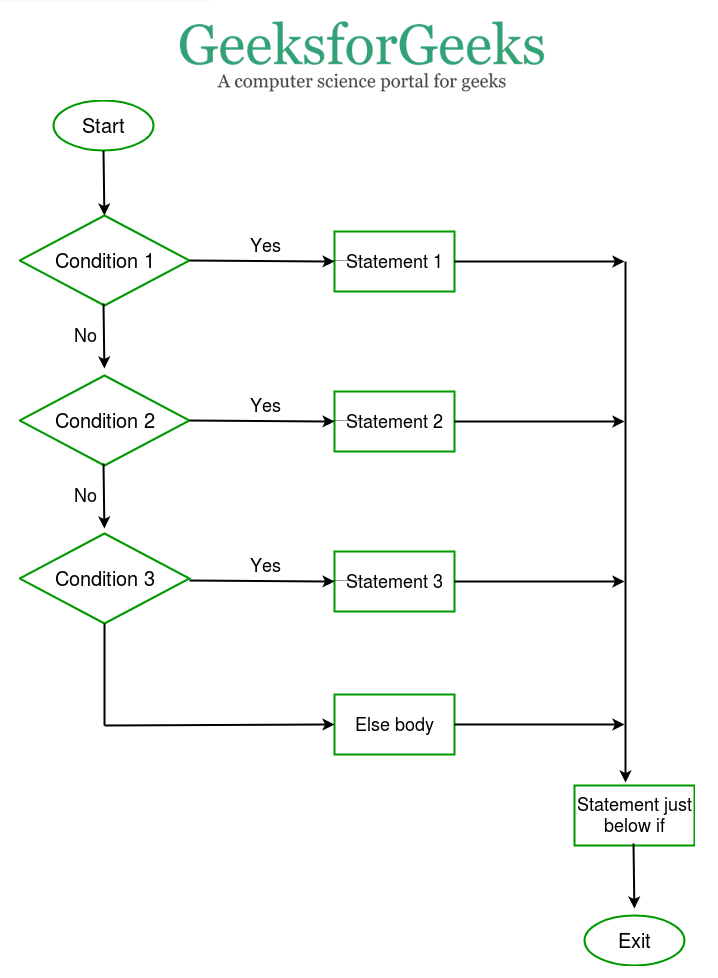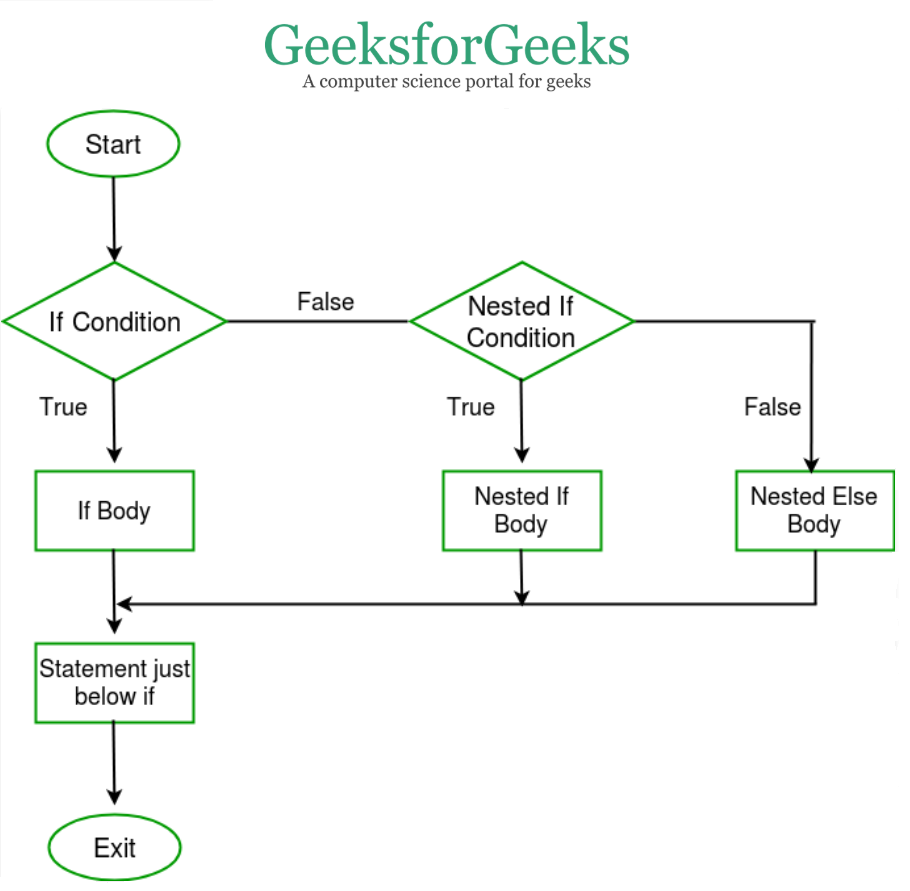# Dart Programming – If Else Statement (if , if..else, Nested if, if-else-if)

Decision-making statements are those statements that allow the programmers to decide which statement should run in different conditions. There are four ways to achieve this:

### if Statement:

This type of statement simply checks the condition and if it is true the statements within it are executed but if it is not then the statements are simply ignored in the code.

`Syntax: if ( condition ){  // body of if}`Example:

## Dart

 `void` `main()` `{` `    ``int` `gfg = 10;`   `    ``// Condition is true` `    ``if` `(gfg > 3) { ` `      ``// This will be printed` `        ``print(``"Condition is true"``); ` `    ``}` `}`

Output:

`Condition is true`

### if…else Statement:

This type of statement simply checks the condition and if it is true, the statements within are executed but if not then else statements are executed.

`Syntax: if ( condition ){  // body of if}else {  // body of else}`Example:

## Dart

 `void` `main()` `{` `    ``int` `gfg = 10;`   `    ``// Condition is false` `    ``if` `(gfg > 30) { ` `      ``// This will not be printed` `        ``print(``"Condition is true"``); ` `    ``}` `    ``else` `{` `      ``// This will be printed` `        ``print(``"Condition id false"``); ` `    ``}` `}`

Output:

`Condition is false`

This type of statement simply checks the condition and if it is true the statements within it are executed but if it is not then other if conditions are checked, if they are true then they are executed, and if not then the other if conditions are checked. This process is continued until the ladder is completed.

`Syntax: if ( condition1 ){  // body of if}else if ( condition2 ){  // body of if}...else {  // statement}`Example:

## Dart

 `void` `main()` `{` `    ``int` `gfg = 10;` `    ``if` `(gfg < 9) {` `        ``print(``"Condition 1 is true"``);` `        ``gfg++;` `    ``}` `    ``else` `if` `(gfg < 10) {` `        ``print(``"Condition 2 is true"``);` `    ``}` `    ``else` `if` `(gfg >= 10) {` `        ``print(``"Condition 3 is true"``);` `    ``}` `    ``else` `if` `(++gfg > 11) {` `        ``print(``"Condition 4 is true"``);` `    ``}` `    ``else` `{` `        ``print(``"All the conditions are false"``);` `    ``}` `}`

Output:

`Condition 3 is true`

## Nested if Statement:

This type of statement checks the condition and if it is true then the if statement inside it checks its condition and if it is true then the statements are executed otherwise else statement is executed.

`Syntax: if ( condition1 ){  if ( condition2 ){     // Body of if  }  else {    // Body of else  }}`Example:

## Dart

 `void` `main()` `{` `    ``int` `gfg = 10;` `    ``if` `(gfg > 9) {` `        ``gfg++;` `        ``if` `(gfg < 10) {` `            ``print(``"Condition 2 is true"``);` `        ``}` `        ``else` `{` `            ``print(``"All the conditions are false"``);` `        ``}` `    ``}` `}`

Output:

`All the conditions are false`

Try code of if-else with Operator:

## Dart

 `void` `main() {` `  `  `  ``int` `x = 5;` `  ``int` `y = 7;` `  `  `  ``if``((++x > y--) && (++x < ++y)){` `      ``print(``"Condition true"``);` `  ``}` `  ``else` `{` `      ``print(``"Condition false"``);` `  ``}` `  ``print(x);` `  ``print(y);` `}`

`Output:Condition false66`

Whether you're preparing for your first job interview or aiming to upskill in this ever-evolving tech landscape, GeeksforGeeks Courses are your key to success. We provide top-quality content at affordable prices, all geared towards accelerating your growth in a time-bound manner. Join the millions we've already empowered, and we're here to do the same for you. Don't miss out - check it out now!

Previous
Next